# Map and analyze raster data in R

The amount of spatial analysis functionality in R has increased dramatically since the first release of R. In a previous post, for example, we showed that the number of spatial-related packages has increased to 131 since the first R release. This means, of course, that more and more of your spatial-related workflow can be conducted without leaving R.

In this post we show some simple (and not-so-simple) examples of how to work with raster data in R with a focus on the `raster` package. This post also makes extensive use of the “new” R workflow with the packages `dplyr`, `magrittr`, `tidyr` and `ggplot2`.

We will load the key libraries. If you're unfamiliar with `dplyr` and `tidyr`, which we use for data processing, you can check out our previous post on the topic. Be sure to load `tidyr` before `raster`, otherwise the `extract` tool from the raster library will be masked.

``````# data processing
library(dplyr)
library(magrittr)
library(tidyr)
library(ggplot2)
library(gridExtra) # to arrange grid plots

# spatial
library(raster)
library(rasterVis)
library(rgdal)
library(dismo) #map raster on Google Map
``````

QGIS has a useful selection of sample data on their website. The data includes two raster datasets as well as multiple vector datasets. Careful, the size of the zipped file is approximately 21 Mbs. In our example, we are putting the data in a temporary folder which we’ve hard coded. You could alternatively do this with the `tempdir` function.

``````# data location

mydir<-"D:\\junk"
temp<-tempfile(tmpdir=mydir, fileext=".zip")
unzip(temp, exdir=mydir)

# Grab the name of the file path
fpath<-list.files(path = mydir, full.names = TRUE, pattern = "qgis_sample_data")
fpath<-gsub("/", "\\\\", fpath)
``````

### 3. Read in and reclassify the raster data

We often need to tabulate the area of different types of land cover (from a raster) by a region (like census regions). We will show you how to do this with the `raster` package and then compare the results with ESRI's Tabulate Area tool. In this example we're using the AVHRR Global Land Cover Classification image that comes with QGIS sample data and we’re using the regions shapefile from QGIS. The land cover raster is a little old – it's good for a demonstration, not so good if you’re interested in current land cover. Note that to save typing we call land cover “land use” in the code.

``````# Read in landcover raster
landusepath<-paste(fpath, "raster\\landcover.img", sep="\\")
landuse.raw<-raster(landusepath)
plot(landuse.raw, axes=FALSE)
``````

There are 14 possible land cover categories but for simplicity in this example, I'm going to limit my data to water (raster value 0), roughly green areas (1-8, 10,11), shrubland (9) and urban (13). This is a very rough categorization, if you want to make changes you can take a look at the categories here (this data is 1KM resolution). To do the reclass I'm using the `reclassify` function and with, as input, a matrix of two columns with the first as “to”, and the second as “becomes”. I'll label water, green, shrub and urban as 0, 1, 9, 13 respectively and then map the reclassified raster.

``````vals<-unique(values(landuse.raw))
recl<-matrix(c(vals, c(0, rep(1, 6), 9, 1,1, 13)),ncol=2)
recl
##       [,1] [,2]
##  [1,]    0    0
##  [2,]   12    1
##  [3,]    9    1
##  [4,]    8    1
##  [5,]   10    1
##  [6,]    7    1
##  [7,]    6    1
##  [8,]    1    9
##  [9,]    5    1
## [10,]    4    1
## [11,]   13   13

landuse<-reclassify(landuse.raw, rcl=recl)
plot(landuse, legend=FALSE, axes=FALSE)
``````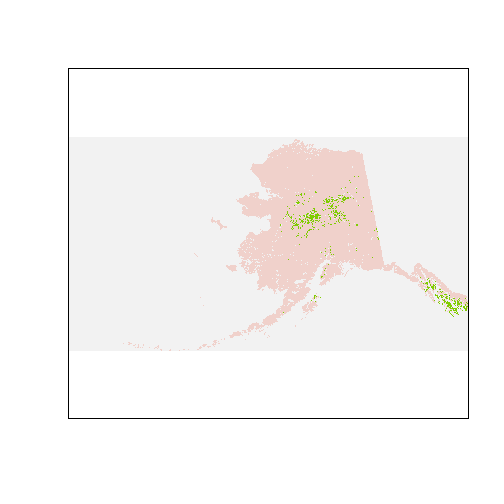This is significantly simpler — just four categories to work with.

### 4. Read in and map the region data

We pulled in the raster data using the `raster` function and now we will read in the polygon data using `readOGR` from the `rgdal` package. We prefer `readOGR` because, unlike the `readShapePoly` in `maptools`, it reads in the projection information by default. We then use the package `ggplot2` to map the data. We have a post on mapping in ggplot2 if you’d like more information.

``````# Regions polygon shapefile
regionpath<-paste(fpath, "shapefiles", sep="\\")

# we will use ggplot to plot the regions
ggplot()+geom_polygon(data=region,  aes(x=long, y=lat, group=group),
coord_equal()+xlim(c(-5000000, 5000000))+ylim(c(1000000, 8000000))
``````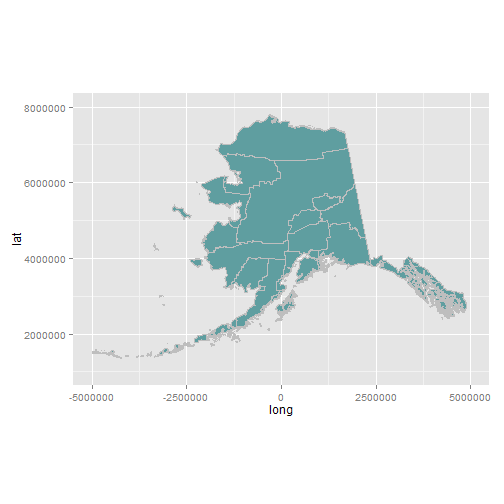So far so good.

### 5. Filter/clip our geographic data to our regions of interest

We're interested in land cover types in three regions of Alaska. Our first step is to filter the regions (the polygons) to our regions of interest and then clip the raster to match. If all you care about are land cover tabulations by region you actually do not have to clip the raster but since we want a map that displays only our three regions we need to clip. There is a nice answer on stackexchange by Jeffrey Evans on clipping a raster that I borrow from.

To “clip” the raster we first crop the raster to the extent of the three regions, then use the function `rasterize` to create a raster version of the regions and finally use that region-raster to clip, or in raster-speak, `mask` the land cover raster.

``````
# Create a subset with our regions of interest
myregions<-c( "Anchorage", "Yukon-Koyukuk", "North Slope")
region.sm<-region[region\$NAME_2 %in% myregions,]

cr<-crop(landuse, region.sm)
fr<-rasterize(region.sm, cr)

# let's map those pieces so you can see the result. Since I just
# want the raster with no legend/axes etc I'm creating a function
# to strip the plot

nakedMap<-function(dat, title=""){
gplot(dat)+geom_tile(aes(fill=value))+
ggtitle(title)+
coord_equal()+
scale_x_continuous(expand = c(0,0)) +
scale_y_continuous(expand = c(0,0)) +
theme(line = element_blank(),
line = element_blank(),
axis.text=element_blank(),
axis.title=element_blank(),
legend.position = "none")
}

cr.plot<-nakedMap(cr, title="Cropped")
fr.plot<-nakedMap(fr, title="Regions")

grid.arrange(cr.plot, fr.plot, lr.plot, ncol=3) #use package gridExtra
``````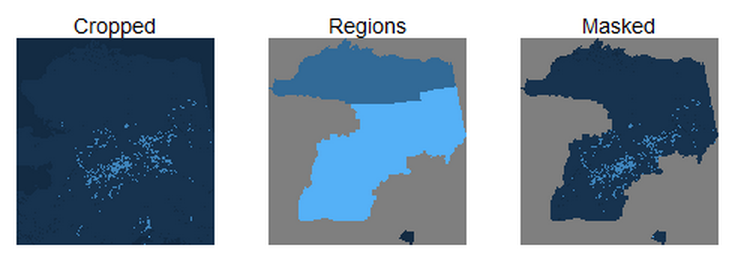Great! Now that we have the pieces we need we're ready to make a nicer map of land cover in our three regions.

### 6. Make a nicer map

To make a nice map of the regions I'm going to use a function called `gplot` from the `rasterVis` package by Oscar Perpiñán. Careful, we're using `gplot` (one 'g') not `ggplot`. The `gplot` function is a wrapper around the `ggplot2` package – essentially allowing us to use `ggplot2` methods with raster data.

``````# centroids for the labels
centroids<-cbind(coordinates(region.sm), region.sm@data)
names(centroids)[1:2]<-c("x", "y")

# use gplot (not ggplot) from rasterVis
# geom_text adds the labels at the centroids
gplot(lr)+
geom_tile(aes(fill=factor(value, labels=c("Water", "Green", "Shrubland", "Urban"))), alpha=0.8)+
scale_fill_manual(values = c("steelblue3", "forestgreen", "ghostwhite", "red"),
name= "Land use code")+
geom_polygon(data=region.sm, aes(x=long, y=lat, group=group),
fill=NA,color="grey50", size=1)+
geom_text(data=centroids, aes(x=x, y=y, label=NAME_2), fontface="bold")+
coord_equal()
``````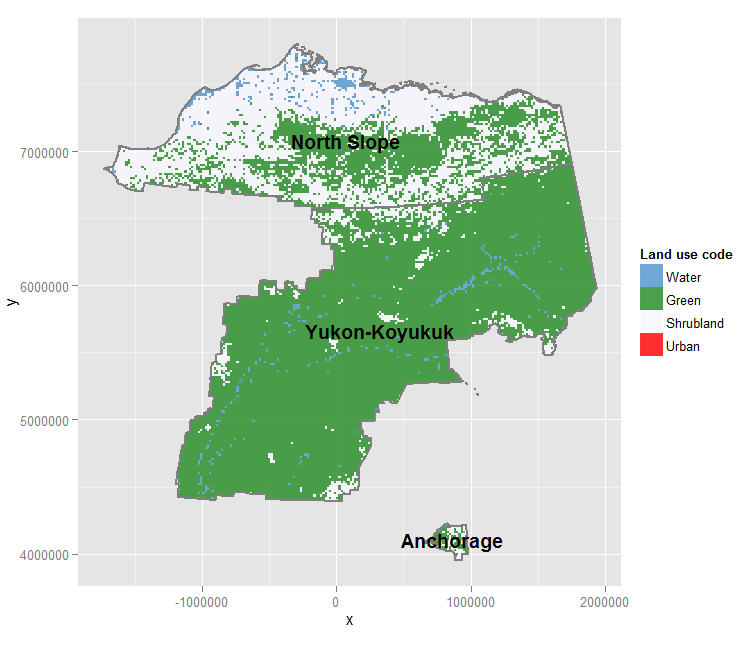Looks nice, there are clear trends in green and shrubland, in particular. There is very little urban land so it’s basically not visible (if you look really, really closely you’ll see a tiny bit of red in Anchorage). Now let's tabulate areas by type of land cover.

### 7. Extract land cover values by region and tabulate

We will use the `extract` function from the `raster` package to grab land cover values by region and tabulate. I mentioned above that we can actually perform this on the original raster of Alaska and the code is below. But since we have a clipped raster we may as well use this.

``````# Extract the values of the landcover raster for each zone.
# This produces a list of raster cells for each region

# You can do the same calculation using the full state raster
# ext<-extract(raster, region.sm, method='simple')

# this takes a little time
ext<-extract(lr, region.sm, method='simple')
class(ext)  # a list
##  "list"
length(ext) # three elements, a vector of land use values for each region
##  3

# Function to tabulate land use by region and return
# a data.frame
tabFunc<-function(indx, extracted, region, regname) {
dat<-as.data.frame(table(extracted[[indx]]))
dat\$name<-region[[regname]][[indx]]
return(dat)
}

# run through each region and compute a table of the count
# of raster cells by land use. Produces a list (see below)
tabs<-lapply(seq(ext), tabFunc, ext, region.sm, "NAME_2")
tabs
## []
##   Var1 Freq      name
## 1    0  241 Anchorage
## 2    1 4260 Anchorage
## 3   13   37 Anchorage
##
## []
##   Var1   Freq        name
## 1    0   8819 North Slope
## 2    1 226463 North Slope
##
## []
##   Var1   Freq          name
## 1    0   6069 Yukon-Koyukuk
## 2    1 347948 Yukon-Koyukuk
## 3    9  27052 Yukon-Koyukuk

# assemble into one data frame
tabs<-do.call("rbind",tabs )

# name the land uses
tabs\$Var1<-factor(tabs\$Var1, levels=c(0,1,9,13), labels=c("Water", "Green", "Shrubland", "Urban"))

# use the spread function from tidyr to make nicer
tabs%>%
group_by(name) %>% # group by region
mutate(totcells=sum(Freq), # how many cells overall
percent.area=round(100*Freq/totcells,2)) %>% #cells by landuse/total cells
dplyr::select(-c(Freq, totcells)) %>% # there is a select func in raster so need to specify
spread(key=Var1, value=percent.area, fill=0) # make wide format
## Source: local data frame [3 x 5]
##
##            name Water Green Shrubland Urban
## 1     Anchorage  5.31 93.87       0.0  0.82
## 2   North Slope  3.75 96.25       0.0  0.00
## 3 Yukon-Koyukuk  1.59 91.31       7.1  0.00
``````

We can see that the Anchorage region is the only one with urban land cover (though very little of it) and, as you can see in the maps, the North Slope has the highest percentage of shrubland and Yukon-Koyukuk has the most green area.

### 8. Compare with ArcGIS's Tabulate Area tool results

Since most of us started doing these kinds of calculations in ArcGIS it may be comforting to see the R results side-by-side with those from ArcGIS – in particular the tabulate area tool.

In order to follow along on your own computer you need to have ArcGIS installed and Python needs to be accessible on the command line. We don't want to leave the comforts of R so here we will create a python script from within R (using the `cat` function) and then we will run it with the `system` function.

By the way, if someone wants to submit the code for doing this in QGIS, I will add it here with attribution.

``````
scriptfile<-paste0(mydir, "\\tabulate_area.py")
outfile<-paste0(mydir, "\\out.dbf")

# NOTE 1: encode string to keep the double backslashes for Python
# NOTE 2: ArcGIS is finicky. TabulateArea requires an integer or
# string as the zone so we create a new field of "Value" as an integer

# cat creates an external file
cat(paste0("import arcpy
arcpy.env.overwriteOutput = True
arcpy.CheckOutExtension('Spatial')
regions = '", encodeString(paste0(regionpath, "\\regions.shp")), "'
landcover = '", encodeString(landusepath), "'
outtable = '", encodeString(outfile), "'
arcpy.CalculateField_management(landcover, 'value2', '!Value!','PYTHON_9.3')
arcpy.gp.TabulateArea_sa(regions, 'NAME_2', landcover, 'value2', outtable)
"), file=scriptfile)

system(paste("python", scriptfile))
``````

So that we have a pretty plot with more than just three regions, let's calculate the total area of each land cover for all regions (not just the three).

``````# pull out land uses by region into a list
# this takes a minute or so on my machine
extall<-extract(landuse.raw, region, method='simple')

# run the function to tally land uses and create table
tabsall<-lapply(seq(extall), tabFunc, extall, region, "NAME_2")
tabsall<-do.call("rbind", tabsall)

# so that we can join more easily convert factors to character
tabsall %<>% mutate(
name=as.character(name),
Var1=as.character(Var1))
``````

Now read in our results from ArcGIS and do some reformating.

``````# read in our table
head(arcgis[,1:5]) #take a look at 1st five cols
##           NAME_2     VALUE_0    VALUE_1  VALUE_4    VALUE_5
## 1 Aleutians East 26713107200   96825600        0          0
## 2 Aleutians West 49499398400          0        0          0
## 3      Anchorage  2635808000          0        0          0
## 4         Bethel 98762112000 2711116800        0  602470400
## 5    Bristol Bay  1334041600          0        0          0
## 6         Denali   892947200 6239872000 21516800 1366316800

# convert to long format to match our other data
arcgis%<>%gather(key=Var1, value=area, -NAME_2)%>%
rename(name=NAME_2) %>%
mutate(Var1=gsub("VALUE_", "", Var1),
name=as.character(name))

# join the two tables
# note that arcgis returns 0 for land uses that do not occur
# in a region while in the raster packages no cells of this
# type occur so with a full join you get 0 for Arc and NA for R.

both<-full_join(tabsall, arcgis, by=c("name", "Var1")) %>%
mutate(Freq=ifelse(is.na(Freq), 0, Freq))

# the area is in square feet -- to convert to "Cells" which
# are each 1km sq. we need to divide by the number of feet in a
# KM (3280) squared

both%<>%mutate(Freq_GIS=area/(3280^2))
``````

Let's plot and see how they compare:

``````
ggplot(both, aes(Freq, Freq_GIS))+geom_point(size=4,alpha=0.5, color="purple4")+
coord_equal()+geom_abline()+
labs(x="R Raster Package Extract", y="ArcGIS Tabulate Area",
title="Compare R Raster Package Extract\nArcGIS Tabulate Area")
``````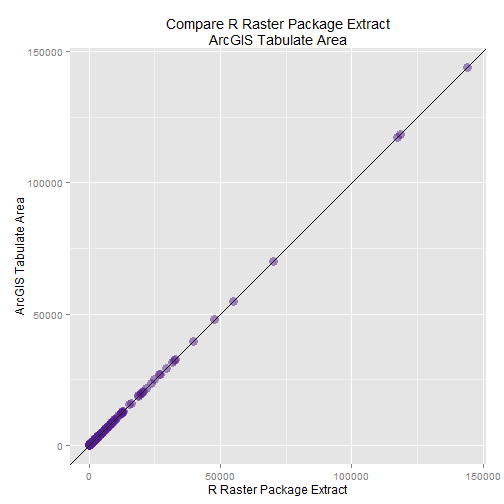Essentially the same results from the two methods.

### 9. Create a RasterBrick and do raster math

As a final step/example we will use the sample data from QGIS to demonstrate how to create a new raster using raster math (similar to raster calculator in ArcGIS). In this example we will identify forest areas with elevations above 200 meters using two input rasters. Here are the steps:

#### a. Read in elevation data

We already have land cover so let’s read in elevation. The QGIS sample data has an elevation raster that we will read.

``````# Elevation raster
elev<-raster(elevpath)
``````

#### b. Make sure both rasters have same cell size and extent

In order to conduct the raster calculations we will create what is called a RasterBrick – essentially a three dimensional raster – but in order to do this we need the two rasters to have the same extent and resolution. We will use the `resample` function to do this.

``````# Are the resolutions the same
res(landuse.raw)
##  3280 3280
res(elev)
##  7181.354 7181.354

# No, we will use resample to make the same resolution
# NOTE: this takes a minute or so to run
elev<-resample(elev, landuse.raw, method="ngb")

# Check again to see that the resolutions match
res(landuse.raw)
##  3280 3280
res(elev)
##  3280 3280

# we manually selected an extent to crop to
ext<-extent(-2500000, 2500000, 2000000, 7650000)

elev<-crop(elev, ext)
landuse.raw<-crop(landuse.raw, ext)
``````

#### c. Create the RasterBrick

A RasterBrick is a multi-layer raster object. As is discussed in the help, a RasterBrick is very similar to a RasterStack (in fact, the calculations below could be done with a RasterStack instead) but processing time may be shorter with a brick at the expense of a little less flexibility.

``````landelev<-brick(landuse.raw, elev)
# to create a RasterStack instead
# stack(landuse.raw, elev)
``````

#### d. Do the raster math

We want to identify areas where the elevation is > 200 and the landcover type is Evergreen Needleleaf Forest or Evergreen Broadleaf Forest (land cover values 1,2). The function `overlay` can be used to do the raster math and create the new raster. Note that this is a different function from the deprecated `overlay` function in the `sp` package.

``````# This is creating a new raster where our first raster
# layer in the brick (x) has a value of 1 or 2 and our
# second layer in the brick (y) is greater than or equal to 200.

elevForest<-overlay(landelev, fun = function(x, y) (x == 1 | x == 2) & y > 200)
``````

This creates a raster of TRUE/FALSE:

``````table(values(elevForest))
##
##   FALSE    TRUE
## 2603589   20739
``````

#### e. Map the raster

We're ready to map the results of our raster math (grid cells that are forest and above 200 meters). In order to do this we will take advantage of a package called `dismo` written by the author of the `raster` package, RJ Hijmans, because it has a nice function called `gmap` that will make it easier to map our raster on a Google Map.

``````# Create our gmap
g<-gmap(x = elevForest, type = "hybrid")

# Reproject our raster so it's the same projection as our gmap().
# NOTE: the default method is bilinear which is not appropriate here
# where we have categorical values
elevForest.prj<-projectRaster(from = elevForest, to=g, method="ngb")

# I want the 0 values to be NA so they don't get mapped
elevForest.prj[elevForest.prj == 0]<-NA
``````
``````plot(g)
plot(elevForest.prj, add = T, legend=FALSE, color="red")
``````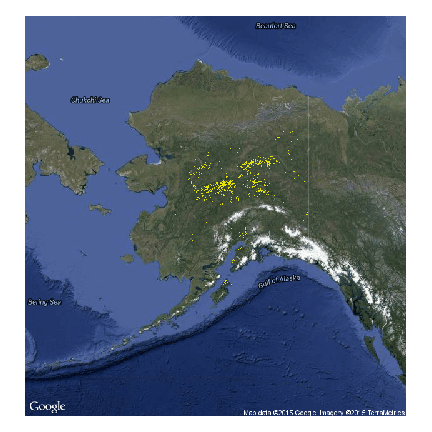And there we have it, forest land above 200 meters elevation computed and mapped in R.

### 10. Conclusion

The amount of spatial functionality in R is incredible. Much of the analysis that used to be done with a traditional GIS can be done in R, significantly simplifying and streamlining analysis workflow. We’ve just touched the surface of analyzing raster data in R in this post. The `raster` and `rasterVis` packages have a ton of functionality that is worth browsing. For interesting additional examples take a look at this page by Oscar Perpiñán.

## 16 responses

1.Faye Gazave says:

Very nice article. Thanks for sharing! I’ve been looking for package that can save 4 dim and 1 dim array data to png. Looking forward to exploring the raster package

2.Martin Montero says:

Thank you very much zevross for sharing your knowledge!
These are beautiful straight examples on how to get the most out of R as a GIS tool.
Everytime I read articles like this, I got more in love of R 🙂

•zev@zevross.com says:

Thank you!

3.Alejandro Coca says:

Zevross, you rock! Thanks for sharing your knowledge in GIS and RS using R.

•zev@zevross.com says:

Thanks!

4.Alejandro Coca says:

Zevross, according to your experience, what is the aim to use the tabulate function with a raster in projected projection in meters if in practical terms you can also estimate area using the number of pixels in the same raster but in geographic projection (e.g. WGS84) and then multiply them with the nominal raster resolution (i.e. 30m for Landsat-based grids)?

•zev@zevross.com says:

With tabulate you don’t need to think as much — as you know, when you move a raster between unprojected and projections your raster cells will change shape and size so you need to be careful using the pixel approach you suggest but, yes, you can do that also in many instances.

5.Ana Calderon says:

Zevross, does this code works well even when some zones/regions overlap each other? I doubled checked the R results against the ArcGIS ones and they are not the same. I know in ArcGIS you can’t have zones that overlap each other. Might this be the reason for discrepancy (i.e. this R code being able to deal with overlapping zones)? Thanks a lot!

•zev@zevross.com says:

That’s a good question we’ve struggled with that issue in ArcGIS before. I don’t know the answer off-hand. Since we’re using an lapply on a region-by-region basis I think this wouldn’t be an issue, but perhaps you can test for me and let me know?

6.Francisco Ollua says:

I got this message, can you help me?
Error in ogrInfo(dsn, layer.ogr) : Cannot open data source
running command ‘”C:\OSGeo4W64\OSGeo4W.bat” “gdal_polygonize” “C:\Users\Francisco\AppData\Local\Temp\Rtmpkt3byn\file31d869292ef.tif” -f “ESRI Shapefile” “C:\Users\FRANCI~1\AppData\Local\Temp\Rtmpkt3byn\file31d8e7d13fc.shp”‘ had status 1

7.Mahrez says:

Thank you very much for this article. It’s very nice and very interessant. However, I have a problem with NA values in step 5, when I masked the raster according to regions :
vals_lr<-unique(values(lr))
NA 0 1 9 13

When I present the map in step 6 (Make a nicer map. However), I have a problem with the "NA" values that located in outside of my cut raster. It display a grey color

I add the option na.value = "transparent" in "scale_fill_manual" to put the NA values in transparency on my map, but the legend of NA always remains !

How to delete in the legend the text "NA" and the box in grey color corresponding?

Is there a solution to remove difinetely the NA values when using the "mask" function or when registering with "Writeraster" to avoid this problem when displaying with ggplot ?

Thank you very much

8.Ludovico says:

Hi, thank you for sharing. I’ve got an issue: when the polygons are larger than the actual extent of the raster (i.e. a regular sized grid that covers the entire raster including nodata areas), the tabFun returns an error which is related with NA’s I suppose

Error in `\$<-.data.frame`(`*tmp*`, name, value = 1L) :
replacement has 1 row, data has 0

•zev@zevross.com says:

You’d probably need to post a reproducible example for me. Put one on stackoverflow.com?

9.Renaud Colmant says:

Great and useful article ! You make it easy to understand and straight to the point. Will start to follow you.

Thanks a lot

10.wilfredbenitez says:

I was extremely pleased to find this great site. I
wanted to thank you for your time just for this wonderful read!!

I definitely really liked every part of it and I have you saved as a favorite
to look at new things on your blog.